# Chemical Tests

GCSEAQAChemistry FoundationChemistry HigherCombined Science FoundationCombined Science Higher

## Chemical Tests

Gasses and ions can be identified using a series of chemical tests. Gasses can be tested for using lime water, flame and pop tests, and litmus paper. Metal ions can be identified using flame tests, giving coloured flames. Metal ions in solutions can be tested for using sodium hydroxide. Carbonate, halide, and sulfate ions can be identified using lime water, silver nitrate, and barium chloride respectively.

## Testing for Gasses

There are multiple gasses that can be produced in chemical reactions that are important to identify. These gasses can be identified using simple test tube chemical reactions. The four most commonly tested for gasses are hydrogen, oxygen, carbon dioxide, and chlorine.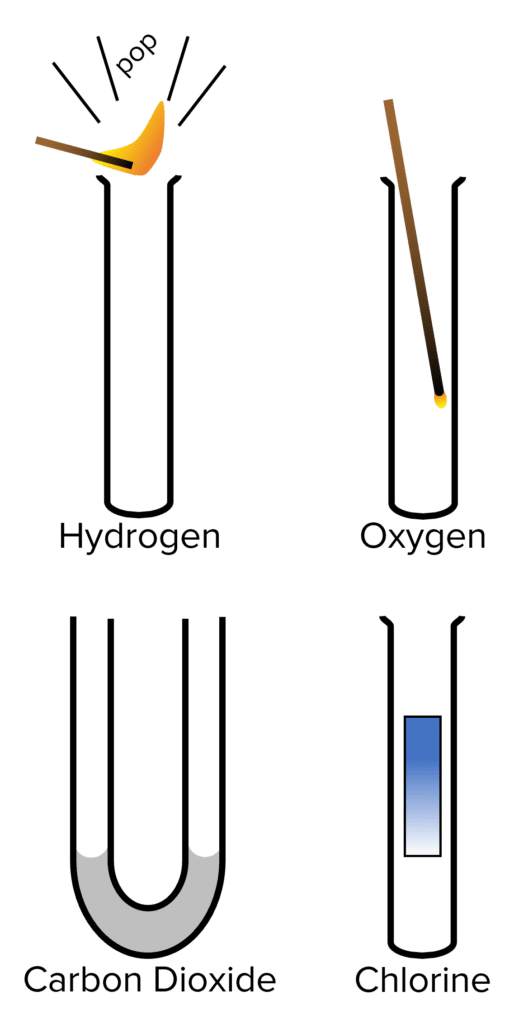Hydrogen

Hydrogen is often given off in reactions between metals and acids. It is a flammable gas and can be tested for with the squeaky pop test.

1. Collect the gas given off by a reaction in a test tube.
2. Take a lit splint and hold it to the mouth of the test tube.
3. If hydrogen is present it will produce a squeaky popping sound.

Oxygen

Often given off by electrolysis, oxygen can be tested for using an embering splint.

1. Collect the gas given off by a reaction in a test tube.
2. Take a lit splint and blow out the flame. Put them embering splint into the test tube.
3. If the flame reignites, oxygen is present.

Carbon Dioxide

Carbon dioxide is given off in a range of reactions. It can be tested for using lime water.

1. Pass the gas given off by a reaction through a U-Tube filled with lime water.
2. If carbon dioxide is present in the gas it, the lime water will become cloudy.

Chlorine

Chlorine is sometimes given off in electrolysis reactions. It can be tested for using damp litmus paper.

1. Collect the gas given off by a reaction in a test tube.
2. Take a piece of damp blue litmus paper and place it in the test tube.
3. If the litmus paper is bleached (i.e. turns from blue to white), chlorine glass is present.
GCSECombined Science FoundationCombined Science HigherChemistry FoundationChemistry HigherAQA

## Metal Ions

Metal ions will can be burnt to produce flames of various different colours. These colours will be characteristic to the metal ion that is burning. These characteristic colours allow us to identify which metal ion is present in a compound by burning a small sample of said compound. Some common ions to test for are lithium, sodium, potassium, calcium, and copper.

Lithium: Burns with a crimson flame.

Sodium: Burns with a yellow flame.

Potassium: Burns with a lilac flame.

Calcium: Burns with an orange/ red flame.

Copper: Burns with a green flame.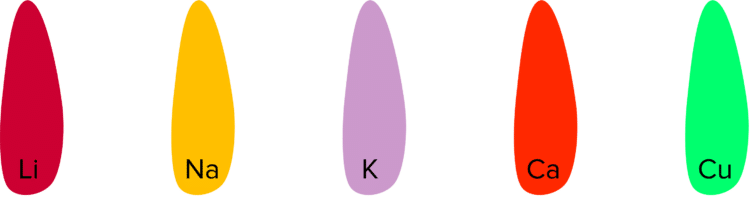The coloured flames of the metal ions mean they can be used to give colour to pyrotechnics. Fireworks are a famous example of the use of metal ions to achieve desired colours from a flame.

GCSEChemistry FoundationChemistry HigherAQA

## Metal Hydroxides

Metal ions can also be identified using sodium hydroxide. Metal ions in solution will often react with sodium hydroxide $\left(\text{NaOH}\right)$ to form metal hydroxide precipitates. The chemical behaviours of these precipitates and their colours will identify the presence of a given ion in solution. Calcium, Aluminium, and Magnesium all form white precipitates upon reaction with $\text{NaOH}$. Aluminium hydroxide can be identified by adding more $\text{NaOH}$ as $\text{Al}\left(\text{OH}\right)_3$ is the only precipitate that will dissolve in excess sodium hydroxide: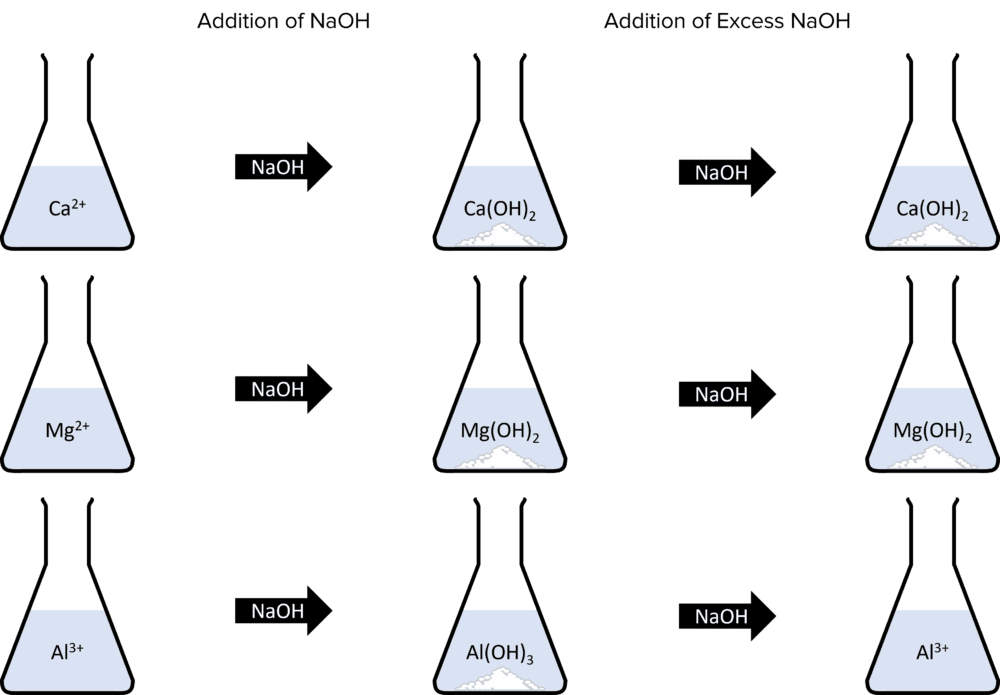The formation of metal hydroxides all follow the same pattern of equations:

$\text{Ca}^{2+}+2\text{NaOH}\rarr\text{Ca}\left(\text{OH}\right)_2+2\text{Na}^{+}$$\\\text{Mg}^{2+}+2\text{NaOH}\rarr\text{Mg}\left(\text{OH}\right)_2+2\text{Na}^{+}$$\\\text{Al}^{3+}+3\text{NaOH}\rarr\text{Al}\left(\text{OH}\right)_3+3\text{Na}^{+}$

When copper and iron ions react with sodium hydroxide, they will produce coloured precipitates. Copper will form a blue precipitate of copper hydroxide and iron forms two precipitates, depending of the charge of the ion. Iron (II) $\left(\text{Fe}^{2+}\right)$ forms a green precipitate and iron (III) $\left(\text{Fe}^{3+}\right)$ forms a brown precipitate: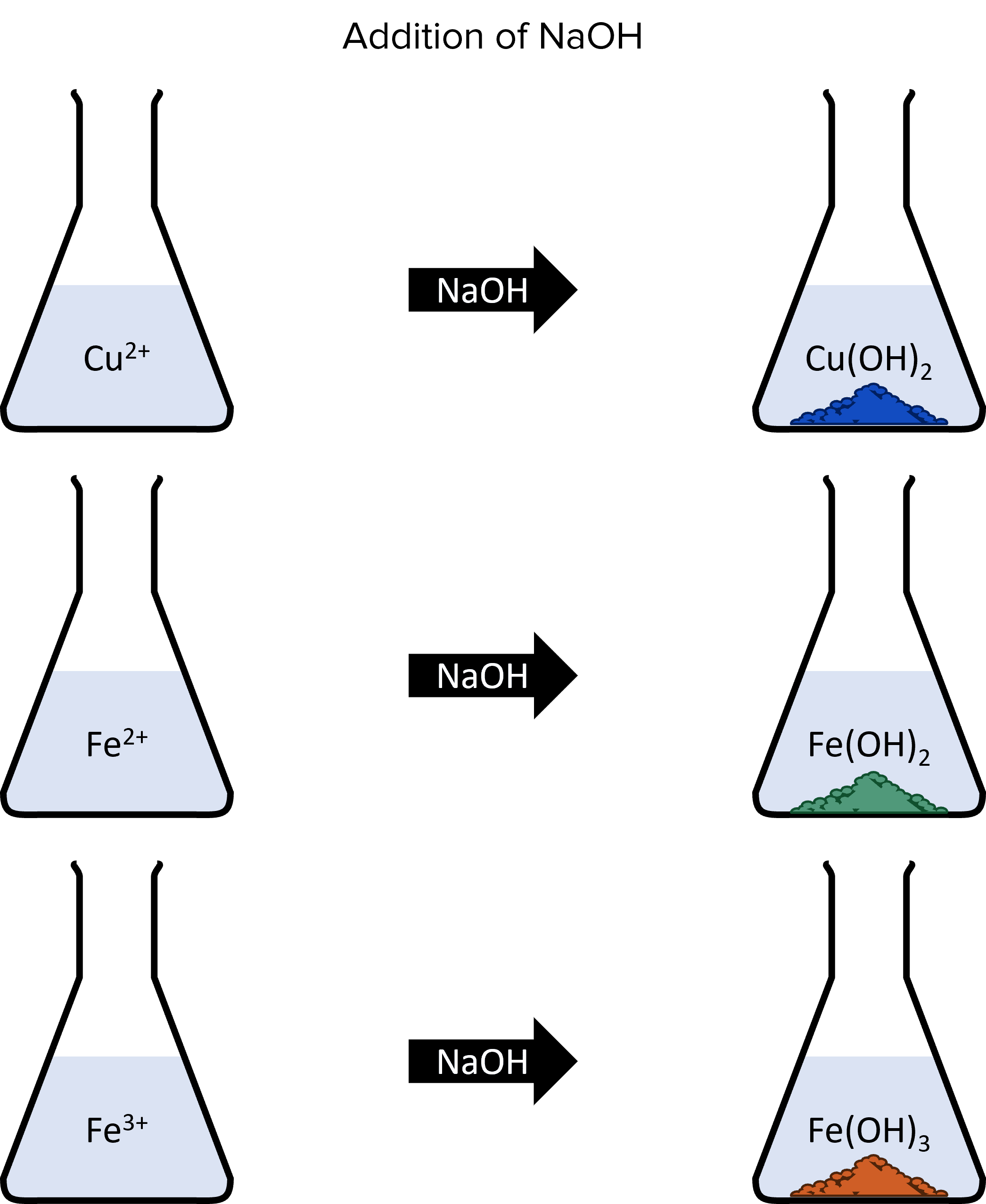Once again, the formation of these precipitates follow the same pattern:

$\text{Cu}^{2+}+2\text{NaOH}\rarr\textcolor{#51997a}{\text{Cu}\left(\text{OH}\right)_2}+2\text{Na}^{+}$$\\\text{Fe}^{2+}+2\text{NaOH}\rarr\textcolor{#134cc0}{\text{Fe}\left(\text{OH}\right)_2}+2\text{Na}^{+}$$\\\text{Fe}^{3+}+3\text{NaOH}\rarr\textcolor{#ce5f26}{\text{Fe}\left(\text{OH}\right)_3}+3\text{Na}^{+}$

GCSEChemistry FoundationChemistry HigherAQA

## Carbonates, Halides, and Sulfates

Carbonate, halide, and sulfate ions are commonly found ions in solutions. They can be tested for using a range of test tube reactions.

Carbonates

Carbonate ions $\left(\text{CO}_3^{ 2-}\right)$ will react with dilute acids. These reactions will produce carbon dioxide gas:

$\text{CO}_3^{ 2-}+2\text{HCl}\rarr\text{H}_2\text{O}+\text{CO}_2+2\text{Cl}^-$

We can test for carbonate ions in a solution by adding dilute hydrochloric acid to the solution and collecting the gas given off. We can then bubble this gas through a sample of lime water. If the lime water turns cloudy, carbon dioxide is present and so carbonate ions were present in the solution.

Halides

Halide ions form when the halogens gain an electron and become negatively charged. Halide ions can be identified by their reactions with aqueous silver nitrate. Chloride, bromide, and iodide ions will all react with silver nitrate to form precipitates. The colours of these precipitates will identify the halide ion that formed them; sliver chloride is a white precipitate, silver bromide is cream, and silver iodide is yellow: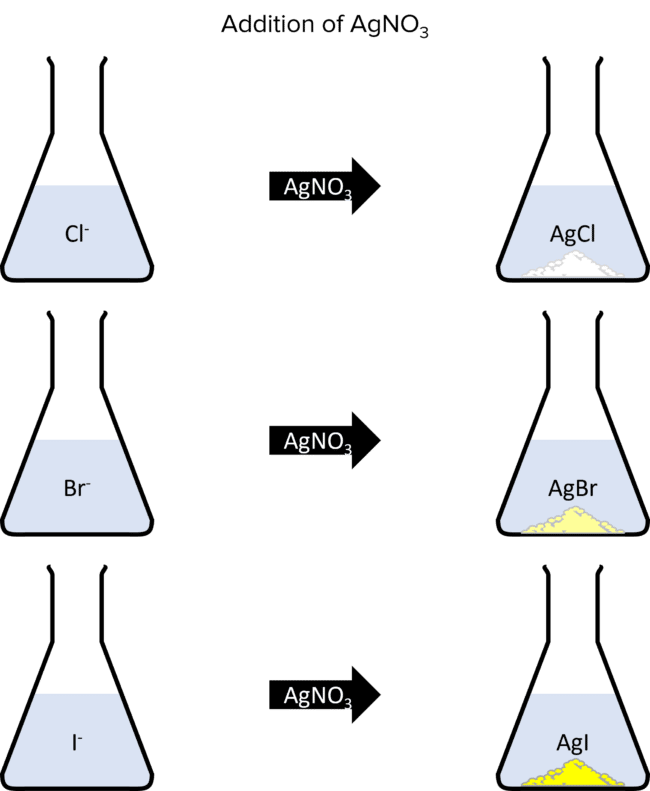These reactions all follow a similar pattern:

\begin{aligned}\text{Cl}^{-}+\text{AgNO}_3&\rarr\text{AgCl}+\text{NO}_3^{ -}\\\text{Br}^{-}+\text{AgNO}_3&\rarr\text{AgBr}+\text{NO}_3^{ -}\\\text{I}^{-}+\text{AgNO}_3&\rarr\text{AgI}+\text{NO}_3^{ -}\end{aligned}

Sulfates

Sulfate ions $\left(\text{SO}_4^{ 2-}\right)$ can be tested for using solutions of a solution of barium chloride mixed with dilute hydrochloric acid. When this mixture is added to a solution that contains sulfate ions a white precipitate of barium sulfate is produced. In this reaction, the larger sulfate ions displace the chloride ions in barium chloride:

$\text{SO}_4^{ 2-}+\text{BaCl}_2\rarr\text{BaSO}_4+2\text{Cl}^{-}$

GCSEChemistry FoundationChemistry HigherAQA

## Identifying Ions

By using a series of chemicals tests, we can identify the ions in a collection of solutions. By using flame tests, we can identify an unknown metal ion.

Flame Test

1. Weigh out $2\text{ g}$ of a metal oxide powder containing and unknown metal ion.
2. Take a splint and use it to scoop up a small amount of the metal oxide powder.
3. Take the splint with the powder and put it into the flame of a Bunsen burner. Record the colour of the flame.
4. Use the colour of the flame to identify the metal ion present in the powder.

Test Tube Reactions

1. Prepare $5$ beakers, each containing a solution with an unknown ion present.
2. From each beaker, take $5$ samples and add each sample to a test tube.
3. Carry out the following test tube reactions using each set of $5$ samples; To the first test tube add an equal volume of sodium hydroxide. To the second, add an excess volume of sodium hydroxide. To the third, add dilute sulfuric acid, collect any gas given off an bubble it through lime water. To the fourth, add a solution of silver nitrate to test for halide. ions. To the fifth, add a solution of barium chloride
4. Record your observations for each test tube in the following table. Use these results to identify which ion is present in each beaker.
 Beaker Observation with $\text{NaOH}$ Excess $\text{NaOH}$ Dilute Acid and Lime Water $\text{AgNO}_3$ $\text{BaCl}_2$ A B C D E
GCSEChemistry FoundationChemistry HigherAQA

## Chemical Tests Example Questions

Carbon dioxide

(Allow $\text{CO}_2$.)Gold Standard Education

Lithium

(Allow $\text{Li}^{+}$.)Gold Standard Education

$\text{Br}^{-}$

(Allow Bromide)Gold Standard Education

Solution: Barium Chloride.

Result: A white precipitate of barium sulfateGold Standard Education

Test tube B contains aluminium hydroxide.

Only aluminium hydroxide dissolves in excess $\text{NaOH}$Gold Standard Education

## Chemical Tests Worksheet and Example Questions

### Tests for Ions and Gases

GCSEOfficial MME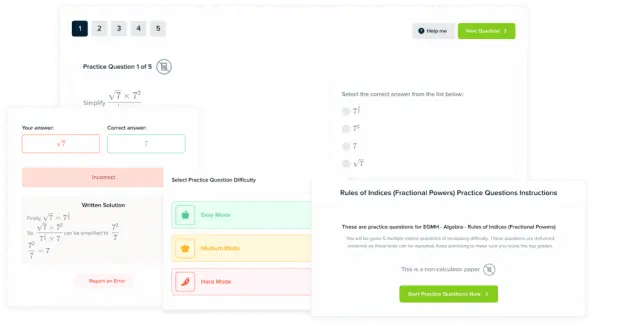Product